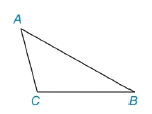Chapter 5.4, Problem 34EElementary Geometry For College St...

7th Edition
Alexander + 2 others
ISBN: 9781337614085

Solutions

Chapter
SectionElementary Geometry For College St...

7th Edition
Alexander + 2 others
ISBN: 9781337614085
Textbook Problem

Given: Δ A B C is not a right Δ Prove: a 2 + b 2 ≠ c 2 (Note: AB = c, AC = b and CB = a.)To determine

To Proof :

c2a2+b2.

Explanation

Given:

ΔABC is not a right Δ

AB=c, AC=b and CB=a.

Approach:

Now a, b and c are the lengths of the three sides of the triangle, with c is the longest side, then

From the triangle theorems,

1. If the square of the length of the longest side is greater than the sum of square of the lengths of other two sides then the triangle is Obtuse.

c2>a2+b2

2. If the square of the length of the longest side is less than the sum of square of the lengths of other two sides then the triangle is Acute.

c2<a2+b2

3. If the square of the length of the longest side is equal to the sum of square of the lengths of other two sides then the triangle is Right triangle.

c2=a2+b2.

So given ABC is not a right triangle then the triangle must be Obtuse or Acute triangle

Still sussing out bartleby?

Check out a sample textbook solution.

See a sample solution

The Solution to Your Study Problems

Bartleby provides explanations to thousands of textbook problems written by our experts, many with advanced degrees!

Get Started

27-30 Find y and y. y=xlnx

Calculus (MindTap Course List)

Identify the four steps of a hypothesis test as presented in this chapter.

Statistics for The Behavioral Sciences (MindTap Course List)

Use the guidelines of this section to sketch the curve. y = arctan(ex)

Single Variable Calculus: Early Transcendentals, Volume I

In Exercises 1124, find the indicated limits, if they exist. 20. limx1x1x1

Applied Calculus for the Managerial, Life, and Social Sciences: A Brief Approach

Factor completely. 2x23x+1

Trigonometry (MindTap Course List)

For the area of the shaded region, which integral form, dx or dy, should be used? a) dx b) dy c) Either...

Study Guide for Stewart's Single Variable Calculus: Early Transcendentals, 8th